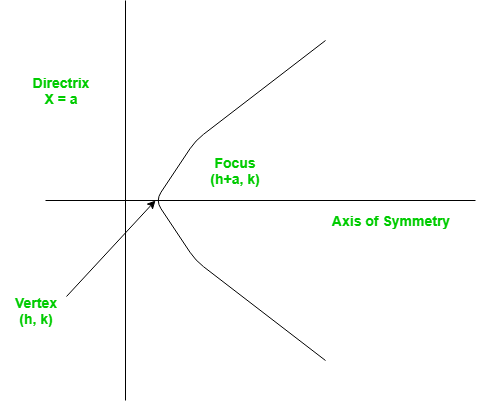# Finding the vertex, focus and directrix of a parabola

Problem – Find the vertex, focus and directrix of a parabola when the coefficients of its equation are given.

A set of points on a plain surface that forms a curve such that any point on that curve is equidistant from the focus is a parabola.
Vertex of a parabola is the coordinate from which it takes the sharpest turn whereas a is the straight line used to generate the curve.The standard form of a parabola equation is. Given the values of a, b and c; our task is to find the coordinates of vertex, focus and the equation of the directrix.

Example –

```Input : 5 3 2
Output : Vertex:(-0.3, 1.55)
Focus: (-0.3, 1.6)
Directrix: y=-198
Consult the formula below for explanation.
```

## Recommended: Please try your approach on {IDE} first, before moving on to the solution.

This problem is a simple example of implementations of formulae. Given below are the required set of formulae which will help us tackle the problem.

```For a parabola in the formVertex:Focus:Directrix:```

## C++

 `#include ` `using` `namespace` `std; ` ` `  `// Function to calculate Vertex, Focus and Directrix ` `void` `parabola(``float` `a, ``float` `b, ``float` `c) ` `{ ` `    ``cout << ``"Vertex: ("` `<< (-b / (2 * a)) << ``", "` `         ``<< (((4 * a * c) - (b * b)) / (4 * a)) ` `         ``<< ``")"` `<< endl; ` `    ``cout << ``"Focus: ("` `<< (-b / (2 * a)) << ``", "` `         ``<< (((4 * a * c) - (b * b) + 1) / (4 * a)) ` `         ``<< ``")"` `<< endl; ` `    ``cout << ``"Directrix: y="` `         ``<< c - ((b * b) + 1) * 4 * a << endl; ` `} ` ` `  `// Driver Function ` `int` `main() ` `{ ` `    ``float` `a = 5, b = 3, c = 2; ` `    ``parabola(a, b, c); ` `    ``return` `0; ` `} `

## Java

 `// Java program to find the vertex, ` `// focus and directrix of a parabola ` ` `  `class` `GFG { ` `     `  `    ``// Function to calculate Vertex,  ` `    ``// Focus and Directrix ` `    ``static` `void` `parabola(``float` `a,  ` `                         ``float` `b, ``float` `c) ` `    ``{ ` `         `  `        ``System.out.println(``"Vertex: ("` `+ ` `                          ``(-b / (``2` `* a)) + ``", "` `+ ` `                          ``(((``4` `* a * c) - (b * b)) / ` `                          ``(``4` `* a)) + ``")"``); ` `                     `  `        ``System.out.println(``"Focus: ("` `+  ` `                          ``(-b / (``2` `* a)) + ``", "`    `+  ` `                          ``(((``4` `* a * c) - (b * b) + ``1``) / ` `                          ``(``4` `* a)) + ``")"``); ` `             `  `        ``System.out.println(``"Directrix:"` `+ ``" y="` `+ ` `                          ``(``int``)(c - ((b * b) + ``1``) *  ` `                          ``4` `* a)); ` `    ``} ` ` `  `    ``// Driver Function ` `    ``public` `static` `void` `main(String[] args) ` `    ``{ ` `        ``float` `a = ``5``, b = ``3``, c = ``2``; ` `         `  `        ``// Function calling ` `        ``parabola(a, b, c); ` `    ``} ` `} ` ` `  `// This code is contributed by  ` `// Smitha Dinesh Semwal `

## Python 3

 `# Function to calculate Vertex,  ` `# Focus and Directrix ` `def` `parabola(a, b, c): ` ` `  `    ``print``(``"Vertex: ("` `, (``-``b ``/` `(``2` `*` `a)), ` `        ``", "``, (((``4` `*` `a ``*` `c) ``-` `(b ``*` `b))  ` `            ``/` `(``4` `*` `a)), ``")"``, sep ``=` `"") ` `               `  `    ``print``(``"Focus: ("` `, (``-``b ``/` `(``2` `*` `a)), ` `    ``", "``, (((``4` `*` `a ``*` `c) ``-` `(b ``*` `b) ``+` `1``) ` `            ``/` `(``4` `*` `a)), ``")"``, sep ``=` `"") ` `                `  `    ``print``(``"Directrix: y="``, c ``-` `((b ``*` `b) ` `                ``+` `1``) ``*` `4` `*` `a, sep ``=` `"") ` ` `  `# Driver Function ` `a ``=` `5` `b ``=` `3` `c ``=` `2` `parabola(a, b, c) ` ` `  `# This code is contributed by Smitha. `

## C#

 `// C# program to find the vertex, ` `// focus and directrix of a parabola ` `using` `System; ` ` `  `class` `GFG { ` `     `  `    ``// Function to calculate Vertex,  ` `    ``// Focus and Directrix ` `    ``static` `void` `parabola(``float` `a,  ` `                         ``float` `b, ``float` `c) ` `    ``{ ` `        ``Console.WriteLine(``"Vertex: ("` `+ ` `                         ``(-b / (2 * a)) + ``", "` `+ ` `                         ``(((4 * a * c) - (b * b)) / ` `                         ``(4 * a)) + ``")"``); ` `                     `  `        ``Console.WriteLine(``"Focus: ("` `+ ` `                         ``(-b / (2 * a)) + ``", "` `+ ` `                         ``(((4 * a * c) - (b * b) + 1) / ` `                         ``(4 * a)) + ``")"``); ` `                 `  `        ``Console.Write(``"Directrix:"` `+ ``" y="` `+  ` `                     ``(``int``)(c - ((b * b) + 1) * 4 * a)); ` `    ``} ` ` `  `    ``// Driver Function ` `    ``public` `static` `void` `Main() ` `    ``{ ` `        ``float` `a = 5, b = 3, c = 2; ` `         `  `        ``// Function calling ` `        ``parabola(a, b, c); ` `    ``} ` `} ` ` `  `// This code is contributed by nitin mittal `

## PHP

 ` `

Output –

```Vertex:(-0.3, 1.55)
Focus: (-0.3, 1.6)
Directrix: y=-198
```

Don’t stop now and take your learning to the next level. Learn all the important concepts of Data Structures and Algorithms with the help of the most trusted course: DSA Self Paced. Become industry ready at a student-friendly price.

My Personal Notes arrow_drop_upCheck out this Author's contributed articles.

If you like GeeksforGeeks and would like to contribute, you can also write an article using contribute.geeksforgeeks.org or mail your article to contribute@geeksforgeeks.org. See your article appearing on the GeeksforGeeks main page and help other Geeks.

Please Improve this article if you find anything incorrect by clicking on the "Improve Article" button below.

Article Tags :
Practice Tags :

Be the First to upvote.

Please write to us at contribute@geeksforgeeks.org to report any issue with the above content.